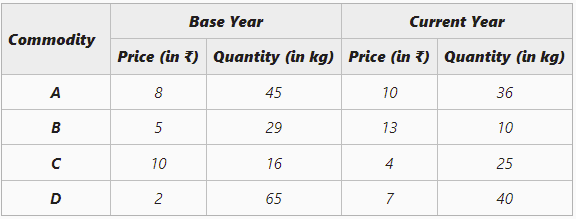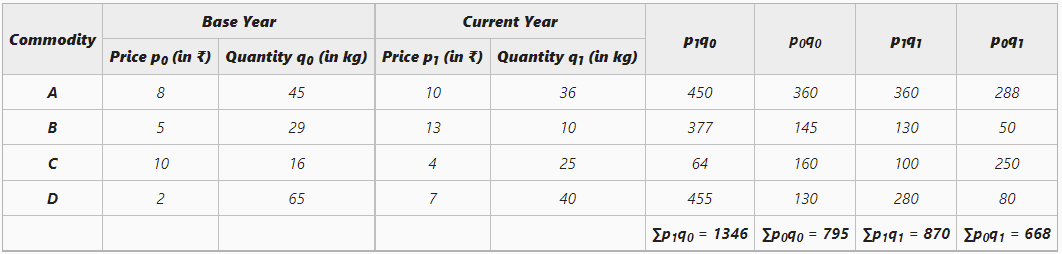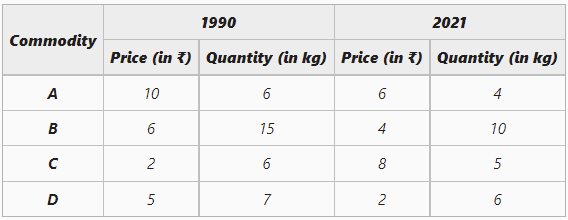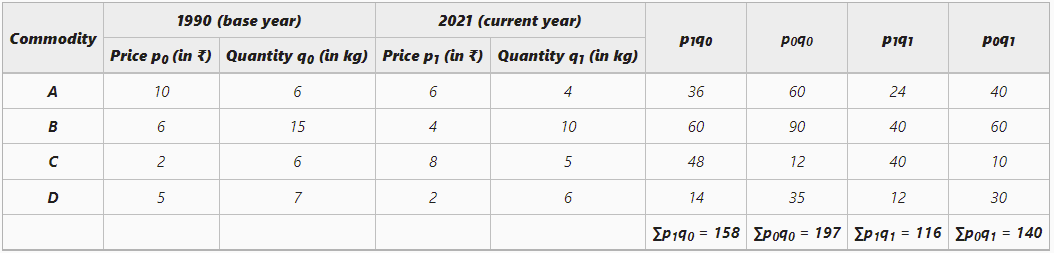GFG App
Open AppBrowser
Continue

# Fisher’s Method of calculating Weighted Index Number

A statistical measure that helps in finding out the percentage change in the values of different variables, such as the price of different goods, production of different goods, etc., over time is known as the Index Number. The percentage change is determined by taking a base year as a reference. This base year is the year of comparison. When an investigator studies different goods simultaneously, then the percentage change is considered the average for all the goods. There are two broad categories of Index Numbers: viz., Simple and Weighted Index Numbers.

According to Spiegel, “An Index Number is a statistical measure designed to show changes in a variable or group of related variables with respect to time, geographic location or other characteristics.”

According to Croxton and Cowden, “Index Numbers are devices for measuring difference in the magnitude of a group of related variables.”

## Fisher’s Method

Weighted Index Numbers can be constructed by using two methods: Weighted Average of Price Relatives Method and Weighted Aggregative Method. Under the Weighted Aggregative Method, different weights are given to different goods according to the quantity bought. There are three methods through which weighted index numbers can be calculated under the Weighted Aggregative Method, viz., Laspeyre’s, Paasche’s, and Fisher’s Method.

The method of calculating Weighted Index Numbers under which the combined techniques of Paasche and Laspeyres are used is known as Fisher’s Method. In other words, both base year and current year’s quantities are used as weights. The formula for Fisher’s Price Index is:Here,

P01 = Price Index of the current year

p0 = Price of goods in the base year

q1 = Quantity of goods in the base year

p1 = Price of goods in the current year

Fisher’s Method is considered the Ideal Method for Constructing Index Numbers.

#### Example 1:

Construct Fisher’s Ideal Index Number using the data given below.#### Solution:= 148.49

The Fisher’s Ideal Index Number is 148.49

#### Example 2

From the following data, construct Fisher’s Index Numbers.#### Solution:= 81.519

The Price Index using Fisher’s Ideal Method is 81.519

My Personal Notes arrow_drop_up